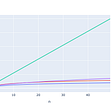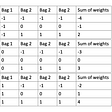# Linear Algebra and Statistics

• Use of vector and matrix notation, especially with multivariate statistics.
• Solutions to least squares and weighted least squares, such as for linear regression.
• Estimates of mean and variance of data matrices.
• The covariance matrix that plays a key role in multinomial Gaussian distributions.
• Principal component analysis for data reduction that draws many of these elements together.

# Vector Spaces

• An operation called vector addition that takes two vectors v, w ∈ V , and produces a third vector, written v + w ∈ V .
• An operation called scalar multiplication that takes a scalar c ∈ F and a vector v ∈ V , and produces a new vector, written cv ∈ V . which satisfy the following conditions (called axioms).
1. Associativity of vector addition: (u + v) + w = u + (v + w) for all u, v, w ∈ V .

# MATRIX TRANSFORMATIONS

--

--

--

## More from Rojinvarghese

Love podcasts or audiobooks? Learn on the go with our new app.

## Statistical Power in Hypothesis Testing## Games To Learn From : How I Beat A National Master (Part 4)## Why computers can’t represent 0.3 accurately## To Stock or Not to Stock## Determine Weight of Counterfeit coins [Asked in GS Interview]## Learn to Love Brackish## Variables UE5